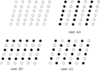International
Tables for
Crystallography
Volume A
Space-group symmetry
Edited by Th. Hahn

International Tables for Crystallography (2006). Vol. A. ch. 9.3, pp. 758-759

## Section 9.3.6. Sublattices

B. Grubera

aDepartment of Applied Mathematics, Faculty of Mathematics and Physics, Charles University, Malostranské nám. 25, CZ-11800 Prague 1, Czech Republic

### 9.3.6. Sublattices

| top | pdf |

A sublatticeof an n-dimensional lattice L is a proper subset of L which itself is a lattice of the same dimension as L. A sublatticeof L causes a decomposition of the set L into, say, i mutually congruent sublattices,itself being one of them (Fig. 9.3.6.1). The number i is called the index of the sublatticeand indicates how many timesis diluted' with respect to L.Figure 9.3.6.1 | top | pdf |Three possible decompositions of a two-dimensional lattice L into sublattices of index 2.

Sublattices are defined in a natural way in those lattices that have centred conventional cells, being generated by the vertices of these cells (decentring'). They are primitive and belong to the same crystal family as the given lattice. Thus, in the cI, cF, tI, oI, oF, oC, mC and hR 7 lattices, we can meet sublattices of indices 2, 4, 2, 2, 4, 2, 2 and 3, respectively.

Theoretically (though hardly in crystallographic practice), the Bravais type of centred lattices can also be determined by testing all their sublattices with the suspected index and finding in any of these sublattices the Niggli cell.

All sublattices of index i of an n-dimensional lattice L can be constructed by a procedure suggested by Cassels (1971). Ifis a primitive basis of the lattice L then primitive basesof all sublattices of index i of the lattice L can be found by the relationswhere the matrixfulfilsThe numberof these matrices is equal to the number of decompositions of an n-dimensional lattice L into sublattices of index i. To determine this number, it is not necessary to construct explicitly the matrices fulfilling (9.3.6.1). The following formulae (Gruber, 1997b) can be used:

 (i) If, whereis a prime number, then(ii) If(mutually different prime numbers,), we deal with any factoraccording to point (i) and multiply all these numbers to obtain the number.

For example, forand i = 2, 3, 4 and 6, we obtain forthe values 7, 13, 35 and 91, respectively.

In all considerations so far, the symmetry of the lattice L was irrelevant. We took L simply as a set of points and its sublattices as its subsets. (Thus, for illustrating sublattices, the triclinic' lattices are most apt; cf. derivative lattices' in Chapter 13.2.)

However, this is not exactly the crystallographic point of view. If, for example, the mesh of the lattice L in Fig. 9.3.6.1were a square, the sublattices in cases (a) and (b) would have the same symmetry (though being different subsets of L) and therefore would be considered by crystallographers as one case only. The numberwould be reduced. From this aspect, the problem is treated in Chapter 13.1in group-theoretical terms which are more suitable for this purpose than the set-theory language used here.

### ReferencesCassels, J. W. S. (1971). An introduction to the geometry of numbers, p. 13. Berlin: Springer.Google ScholarGruber, B. (1997b). Alternative formulae for the number of sublattices. Acta Cryst. A53, 807–808.Google Scholar